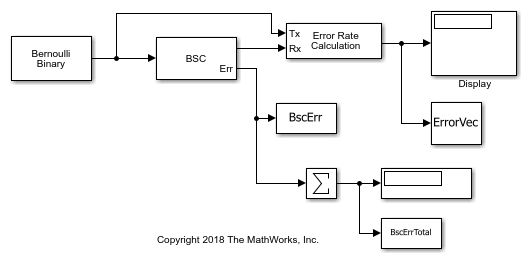# Binary Symmetric Channel Error Port

Pass a random signal through a binary symmetric channel. For a specified error probability, compare the total number of errors indicated by the `Err` port to result reported by the `Error Rate Calculation` block.

Open the `slex_bsc_channel_err` model.Run the model with the error probability of the BSC block set to 0.02. The results from the `Error Rate Calculation` block are saved to the base workspace variable `ErrorVec` in a 1-by-3 row vector. `ErrorVec` contains [ `BER`, `TotalErrors`, `TotalSamplesProcessed` ].

```Total number of errors: 20296 ```

The `Err` port for the `Binary Symmetric Channel` is a binary vector whose length matches the total number of samples processed. By summing the elements of the output `Err` vector you get the total number of errors introduced into the signal data. The sum of the elements of the `Err` vector is saved to the base workspace variable `BscErrTotal`.

```BscErrTotal: 20296 ```

The `Error Rate Calculation` blocks reports the same total number of errors indicated by `BscErrTotal`.